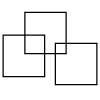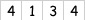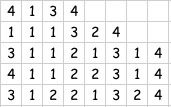#### You may also like### Three Squares

What is the greatest number of squares you can make by overlapping three squares?### Two Dice

Find all the numbers that can be made by adding the dots on two dice.### Biscuit Decorations

Andrew decorated 20 biscuits to take to a party. He lined them up and put icing on every second biscuit and different decorations on other biscuits. How many biscuits weren't decorated?

# Count the Digits

##### Age 5 to 11Challenge Level

We can do all sorts of things with numbers - add, subtract, multiply, divide ...

Most of us start with counting when we are very little. We usually count things, objects, people etc. In this activity we are going to count the number of digits that are the same.

There are a couple of rules about the number we start with:
Rule 1 - The starting number has to have just three different digits chosen from $1, 2, 3, 4$.
Rule 2 - The starting number must have four digits - so thousands, hundreds, tens and ones.
For example, we could choose $2124$ or $1124$.

So when we've got our starting number we'll do some counting. Here is a worked example.

Starting NumberWe then count in order the number of $1$s, then the number of $2$s, then $3$s and lastly $4$s, and write it down as shown here.So the first count gave one $1$, one $3$ and two $4$s.
You may see that this has continued so the third line shows that the line above had three $1$s, one $2$, one $3$ and one $4$.
The fourth line counts the line above giving four $1$s, one $2$, two $3$s and one $4$.

And so it goes on until ... until when?
Your challenge is to start with other four digit numbers which satisfy the two rules and work on it in the way I did.
Tell us what you notice.

What happens if you have five digits in the starting number?